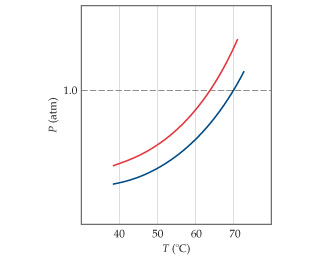# Problem: The following diagram shows the vapor pressure curves of a volatile solvent and a solution of that solvent containing a nonvolatile solute. What are the normal boiling points of the solvent and the solution?

⚠️Our tutors found the solution shown to be helpful for the problem you're searching for. We don't have the exact solution yet.

###### Problem Details

The following diagram shows the vapor pressure curves of a volatile solvent and a solution of that solvent containing a nonvolatile solute.What are the normal boiling points of the solvent and the solution?